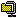### Resource Center

Technical Papers
Working Papers
Research Memoranda
GTAP-L Mailing List
GTAP FAQs
CGE Books/Articles
Important References
Submit New Resource

# GTAP Resources: Resource Display

GTAP Resource #813

"GTAP Model Version 6.1 "
by Hertel, Thomas, Robert McDougall and Ken Itakura

Abstract
This latest release addresses the following 4 bugs found in the previous GTAP.TAB version 6.0 released in June 2001. They are in the equations computing summary variables and may cause errors in the main model when users implement simulations with one of the following specifications:
• Shock the variable DTBALR(r)

• Shock the variable to(ENDW_COMM,r)

• Shock the variable ams(i,s,r)

Bug 1: Computation of DTBARLR

Computation of DTBALR in the equation DTBALRATIO. The equation has been corrected to read:

```Equation DTBALRATIO # change in ratio of trade balance to regional income # (all,r,REG) 100.0 * INCOME(r) * DTBALR(r) = 100.0 * DTBAL(r) - TBAL(r) * y(r);```

Bug 2: Computation of EV_ALT

Computation of EV_ALT in the equation EV_DECOMPOSITION. The modified equation adds the term in bold font as:

```Equation EV_DECOMPOSITION # decomposition of Equivalent Variation # (all,r,REG) EV_ALT(r) = [0.01 * UTILELASEV(r) * INCOMEEV(r)] * ... ... +sum[i,TRAD_COMM,sum(s,REG, VIMS(i,s,r) * ams (i,s,r))] ...```

Bug 3: Computation of Income Tax

Income Tax is now computed separately from indirect taxes. This change affects the equations REGIONALINCOME, and introduces the equation DINDTAXRATIO.

```Equation REGIONALINCOME # regional income = sum of primary factor income and indirect tax receipts# (all,r,REG) INCOME(r) * y(r) = FY(r) * fincome(r) + 100.0 * INCOME(r) * del_indtaxr(r) + INDTAX(r) * y(r) + INCOME(r) * incomeslack(r);```

```Equation DINDTAXRATIO # change in ratio of indirect taxes to INCOME in r # (all,r,REG) del_indtaxr(r) = del_taxrpc(r) + del_taxrgc(r) + del_taxriu(r) + del_taxrfu(r) + del_taxrout(r) + del_taxrexp(r) + del_taxrimp(r);```

Bug 4: GEMPACK "Shock" Statement Bug

This EXE has been made with Release 7.0-003 of GEMPACK. This fixes the bug in which shocks can be misinterpreted by Release 7.0 of GEMPACK. For more information, see Ken Pearson's post dated 9 November 2001.

Resource Details (Export Citation) GTAP Keywords
 Category: Model file (.TAB) Status: Not published By/In: Center for Global Trade Analysis Date: 2001 Version: 6.1 Created: Bacou, M. (9/5/2001) Updated: Bacou, M. (6/18/2002) Visits: 6,581
No keywords have been specified.

Attachments
If you have trouble accessing any of the attachments below due to disability, please contact the authors listed above.

Public AccessGTAP Model files   (1.7 MB)   Replicated: 0 time(s)

Restricted Access
No documents have been attached.

Special Instructions
GTAP.TAB and GTAP.STI files are in this ZIP file.

For RunGTAP extract the contents of this Zip archives in your RunGTAP directory to replace the files.